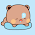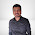Friday, May 28, 2010

MaxFlow :: Dinitz Algorithm

Here is a nice implementation of Dinitz blocking flow algorithm in C++ (with special thanks to Fahim vai). Works in undirected large graph containing multiple edges and self loops as well. No STL used. This implementation is pretty fast.

Here, input is, number of nodes 2≤n≤5000, number of input edges 0≤e≤30000, then e undirected edges in the form (u, v, cap) (1≤u,v≤n and 1≤cap≤10^9). Source and Sink are assumed 1 and n accordingly, can be changed in the init() function call.

`#define SET(p) memset(p, -1, sizeof(p))#define CLR(p) memset(p, 0, sizeof(p))#define i64 long longconst int INF = 0x7fffffff;const int MAXN = 5005, MAXE = 60006;int src, snk, nNode, nEdge;int Q[MAXN], fin[MAXN], pro[MAXN], dist[MAXN];int flow[MAXE], cap[MAXE], next[MAXE], to[MAXE];inline void init(int _src, int _snk, int _n) {    src = _src, snk = _snk, nNode = _n, nEdge = 0;    SET(fin);}inline void add(int u, int v, int c) {    to[nEdge] = v, cap[nEdge] = c, flow[nEdge] = 0, next[nEdge] = fin[u], fin[u] = nEdge++;    to[nEdge] = u, cap[nEdge] = c, flow[nEdge] = 0, next[nEdge] = fin[v], fin[v] = nEdge++;}bool bfs() {    int st, en, i, u, v;    SET(dist);    dist[src] = st = en = 0;    Q[en++] = src;    while(st < en) {        u = Q[st++];        for(i=fin[u]; i>=0; i=next[i]) {            v = to[i];            if(flow[i] < cap[i] && dist[v]==-1) {                dist[v] = dist[u]+1;                Q[en++] = v;            }        }    }    return dist[snk]!=-1;}int dfs(int u, int fl) {    if(u==snk) return fl;    for(int &e=pro[u], v, df; e>=0; e=next[e]) {        v = to[e];        if(flow[e] < cap[e] && dist[v]==dist[u]+1) {            df = dfs(v, min(cap[e]-flow[e], fl));            if(df>0) {                flow[e] += df;                flow[e^1] -= df;                return df;            }        }    }    return 0;}i64 dinitz() {    i64 ret = 0;    int df;    while(bfs()) {        for(int i=1; i<=nNode; i++) pro[i] = fin[i];        while(true) {            df = dfs(src, INF);            if(df) ret += (i64)df;            else break;        }    }    return ret;}int main() {    int n, e, u, v, c, i;    scanf("%d%d", &n, &e);    init(1, n, n);    for(i=0; i<e; i++) {        scanf("%d%d%d", &u, &v, &c);        if(u!=v) add(u, v, c);    }    printf("%lld\n", dinitz());    return 0;}`

Using adjacency matrix and/or STL makes it 10 to 4 times slower.

1.Who knows, how many different ways can a graph be represented...

2.Infinite ways !

3.yeah... they may be lots of ways...

4.what does FIN [] array represent??

1.I maintain the graph as a list of edges where edges from same nodes are maintained similar to a linked list. fin[u] is the final (tail) of one such link. It stores the index of last edge which starts from node u;

5.Do you use the concept of level graph and blocking flow in this implementation? If yes, in which part? Thanks a lot.

6.Could u please explain everything step by step.

7.What is the use of pro[] array?

1.as you can see, the graph is stored in a form of edge list (linked list) here. the list is maintained in a way that all the edges coming out of a specific node can be traversed by using the next[] links. here pro[] holds the heads of each linked list temporarily. the heads can be found in the fin[] array.

8.Can u plzz explain the dfs part of the code ..

9.is it enough to change cap[u][v]=k and cap[v][u]=0 in case of directed to cap[u][v]=k and cap[v][u]=k in undirected .... or there are certain more cases to look(in DINITZ ALGO).

10.does your stl version got ac on SPOJ FASTFLOW?

1.No it got Time limit exceed. I had to use normal array instead of vector, and edge list graph representation instead of adjacency list typically implemented using vector.

11.can u explain why are u adding and subtracting flow at the same time ?
flow[e] += df;flow[e^1] -= df;

1.Yes, that is for balancing augmenting flow.